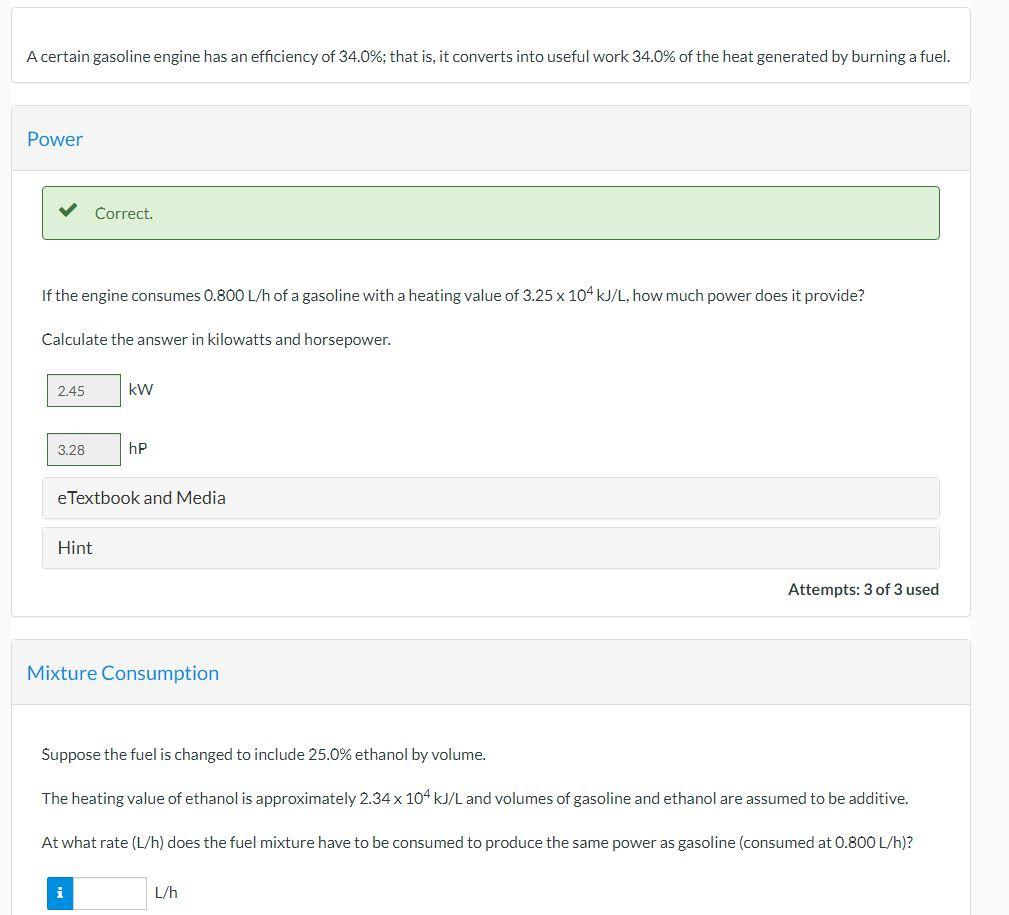# Question Solved1 AnswerA certain gasoline engine has an efficiency of 34.0%; that is, it converts into useful work 34.0% of the heat generated by burning a fuel. Power Correct. If the engine consumes 0.800 L/h of a gasoline with a heating value of 3.25 x 104 kJ/L, how much power does it provide? Calculate the answer in kilowatts and horsepower. 2.45 kW 3.28 hP e Textbook and Media Hint Attempts: 3 of 3 used Mixture Consumption Suppose the fuel is changed to include 25.0% ethanol by volume. The heating value of ethanol is approximately 2.34 x 104 kJ/L and volumes of gasoline and ethanol are assumed to be additive. At what rate (L/h) does the fuel mixture have to be consumed to produce the same power as gasoline (consumed at 0.800 L/h)? L/hTLMSKJ The Asker · Chemical EngineeringTranscribed Image Text: A certain gasoline engine has an efficiency of 34.0%; that is, it converts into useful work 34.0% of the heat generated by burning a fuel. Power Correct. If the engine consumes 0.800 L/h of a gasoline with a heating value of 3.25 x 104 kJ/L, how much power does it provide? Calculate the answer in kilowatts and horsepower. 2.45 kW 3.28 hP e Textbook and Media Hint Attempts: 3 of 3 used Mixture Consumption Suppose the fuel is changed to include 25.0% ethanol by volume. The heating value of ethanol is approximately 2.34 x 104 kJ/L and volumes of gasoline and ethanol are assumed to be additive. At what rate (L/h) does the fuel mixture have to be consumed to produce the same power as gasoline (consumed at 0.800 L/h)? L/h
More
Transcribed Image Text: A certain gasoline engine has an efficiency of 34.0%; that is, it converts into useful work 34.0% of the heat generated by burning a fuel. Power Correct. If the engine consumes 0.800 L/h of a gasoline with a heating value of 3.25 x 104 kJ/L, how much power does it provide? Calculate the answer in kilowatts and horsepower. 2.45 kW 3.28 hP e Textbook and Media Hint Attempts: 3 of 3 used Mixture Consumption Suppose the fuel is changed to include 25.0% ethanol by volume. The heating value of ethanol is approximately 2.34 x 104 kJ/L and volumes of gasoline and ethanol are assumed to be additive. At what rate (L/h) does the fuel mixture have to be consumed to produce the same power as gasoline (consumed at 0.800 L/h)? L/h